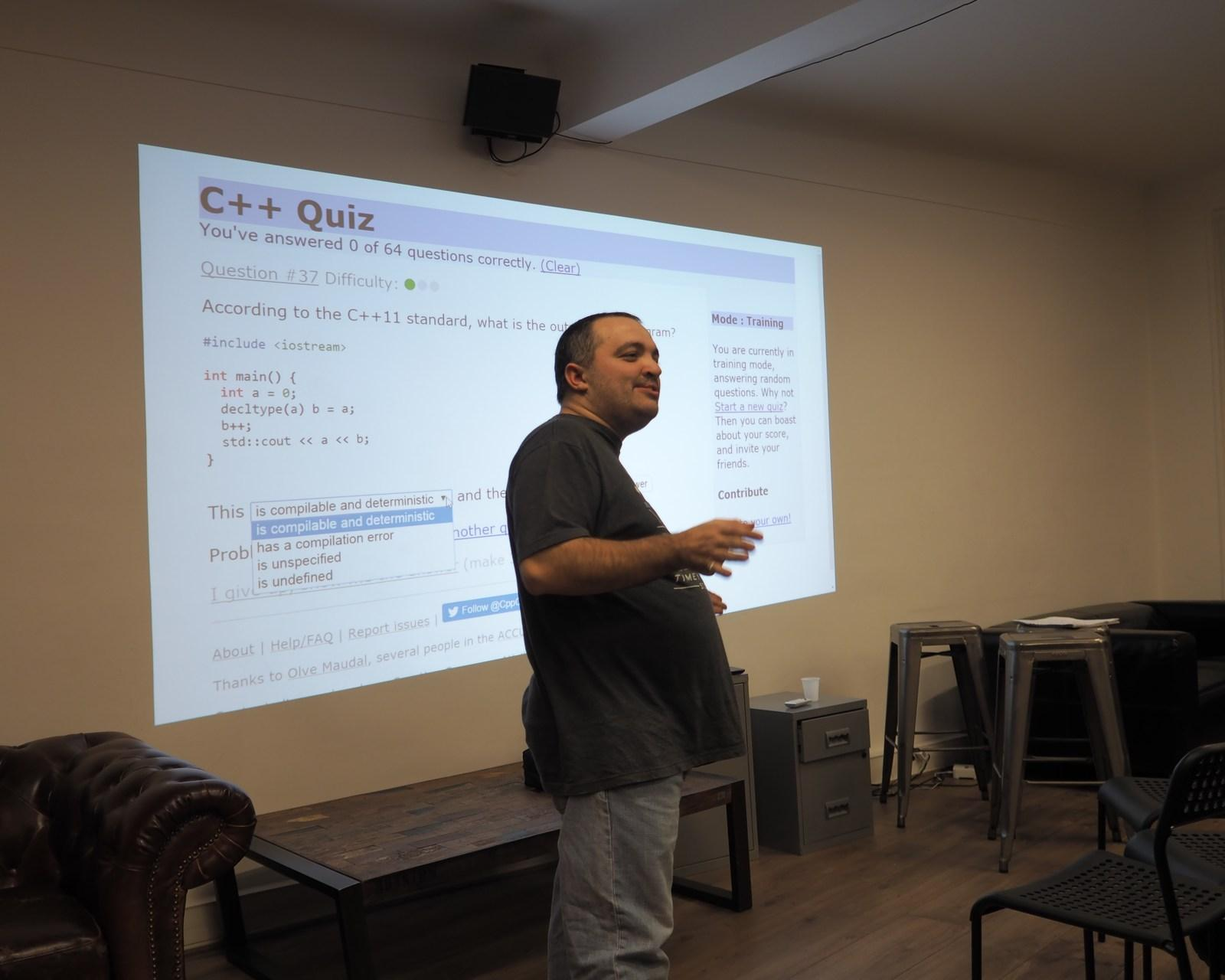# C++ Quiz (1)

## Test your knowledge of the C++ programming language with this quiz! See if you can answer all 12 questions correctly

Next »
1/12

### Which of the following is the correct syntax for declaring a variable in C++?var myVariable;
int myVariable;
myVariable = int;
myVariable = 10;
2/12

### What is the output of the following code?#include <iostream>using namespace std;int main() { int x = 10; cout << x << endl; return 0;}0
10
x
Error
3/12

### What is the correct way to pass arguments by reference in C++?

pass-by-reference
pass-by-pointer
pass-by-value
pass-by-reference using '&'
4/12

### Which of the following is NOT a C++ keyword?break
print
continue
return
5/12

### What does the 'cout' object do in C++?Accepts user input
Displays output to the console
Performs mathematical operations
Stops the program execution
6/12

### What is the correct way to include the 'iostream' library in C++?#include <iostream>
#include iostream
using namespace std;
#include <iostream.h>
7/12

### Which of the following is a valid way to comment a single line in C++?/* Comment */
// Comment
:: Comment
" Comment
8/12

### What is the correct way to declare a function in C++?function myFunction()
myFunction()
void myFunction()
declare myFunction()
9/12

### What is the result of the following expression?5 + 3 * 2 / 44
7
9
5
10/12

### Which of the following is a loop control statement in C++?for
switch
if
class
11/12

### What is the correct way to initialize an array in C++?int myArray[] = {1, 2, 3};
myArray = {1, 2, 3};
int myArray = {1, 2, 3};
int myArray = {1, 2, 3};
12/12

### What is the purpose of the 'cin' object in C++?

Displays output to the console
Performs mathematical operations
Accepts user input
Stops the program execution